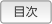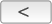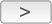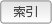Cakewalk // Documentation // SONAR LE // 機能比較表製品の機能比較 SONAR LE SONAR X3 Essential SONAR X3 Studio SONAR X3 Producer 一般 最大オーディオトラック数 32 制限なし 制限なし 制限なし 最大MIDIトラック数 64 制限なし 制限なし 制限なし 最大ビット数/サンプルレート 32ビット/96kHz 64ビット/384kHz 64ビット/384kHz 64ビット/384kHz 最大エフェクト数/インストゥルメント 24 / 8 制限なし 制限なし 制限なし 最大同時バス 8 制限なし 制限なし 制限なし 最大オーディオエフェクトセンド（トラック/バスあたり） 5 制限なし 制限なし 制限なし 内部ミキシングエンジン 32ビット 64ビット 64ビット 64ビット Windows x64のサポート ネイティブx64 ネイティブx64 ネイティブx64 ネイティブx64 ステップシーケンサー2.0 x x x ビデオのインポート/エクスポート x x x x GPUアクセラレーション x x x x アルペジエーター x x x FX Chain x x x FX ChainのUIのカスタマイズ x x x 演奏リスト x x x トラックフォルダ x x x クリップのFX欄 x x x ギターのタブ譜とフレットボードビュー x x x グルーブクォンタイズ、反転、ベロシティスケール、タイムにフィット、タップトラックにフィット、および置換 x x x 外部インサート x x AudioSnap 2.0 x x ARA対応 x x x Melodyne essential x x サラウンドサウンド x x SoundCloudの出力 x x x x MusicXMLの出力 x x x オートメーション録音モード タッチのみ タッチのみ x x ProChannel x x ProChannel QuadCurve Zoom x オーディオプラグインエフェクト Alias Factor x x x x Cakewalk Boost 11 x x x Classic Phaser x x x x Compressor/Gate x x x x HF Exciter x x x x Modfilter x x x x Multivoice Chorus/Flanger x x x x Parametric EQ x x x Para-Q x x x x Tempo Delay x x x Sonitus fx: EQ x x x x Sonitus fx: Reverb x x x x Sonitus fx: Compressor x x x Sonitus fx: Delay x x x Sonitus fx: Gate x x x Sonitus fx: Modulator x x x Sonitus fx: Multiband x x x Sonitus fx: Surround x x x Sonitus fx: Surround Compressor x x Sonitus fx: WahWah x x x Sonitus fx:Phase x x x Channel Tools x x TH2 SONAR x x TH2 Producer x TL-64 Tube Leveler x TS-64 Transient Shaper x BiFilter2 x BREVERB SONAR x LP-64 EQ x x LP-64 Multiband x x Nomad Factory Blue Tubes Bundle x x Nomad Factory BlueVerb DRV-2080 x x Nomad Factory Blue Tubes Analog TrackBox x x Perfect Space（32ビットのみ） x x PX-64 Percussion Strip x VX-64 Vocal Strip x MIDIプラグインエフェクト MFX Arpeggiator x x x x MFX Change Velocity x x x x MFX Chord Analyzer x x x x MFX Echo/Delay x x x x MFX Event Filter x x x x MFX Quantize x x x x MFX Transpose x x x x インストゥルメント Cakewalk Sound Center x x x Cakewalk TTS-1 x x x D-Pro LE x DreamStation DXi（32ビットのみ） x x x DropZone x x x Roland Groove Synth x x x Session Drummer 3 x x x Square I x x x x Studio Instruments Suite x x x x Cyclone x x Pentagon I x x PSYN II x x Rapture x Rapture LE x RXP REX Player x x SFZ Sound Font Player x x Z3ta+ Classic x x AAS Lounge Lizard Session SONAR Edition x AAS Strum Acoustic Session SONAR Edition x True Pianos Amber x XLN Audio Addictive Drums x オーディオループ、MIDIクリップ、プロジェクト 500 MB REX Loopmasters x 500 MB REX/WAV Sample Magic x 210 MB WAV XMIX x MIDIグルーブクリップ x チュートリアルプロジェクト x アーティストデモプロジェクト x